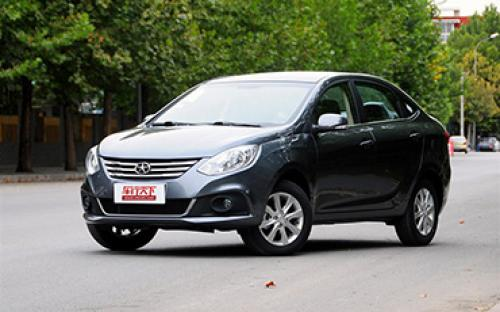江淮 和悦A306 种颜色可选2014款最低售价：5.68 万元起

4435(mm)1725(mm)1505(mm)配置亮点：
• 胎压监测装置

• ISOFIX儿童座椅接口

• 车身稳定控制(ESC/ESP/DSC等)

• 电动天窗

• 定速巡航

• 后倒车雷达

• 真皮座椅

• GPS导航系统

• 氙气大灯

• 后视镜加热江淮 和悦A30 在售车型

排量 车型 厂商指导价 本地最低报价 购车工具
1.5L 1.5L 手动尊享型 5挡手动 6.98万 5.68万
1.30万

询底价+对比

江淮 和悦A30 经销商

查看更多 >>
更多 >>

本地降价排名

江淮 和悦A30 动力加速

和悦A30 0-100公里加速时间分布在 0.0-秒 属于 超跑级

动力级别 加速时间 车型

江淮 和悦A30 视频

江淮 和悦A30 新闻资讯

找到我的经济适用女 淮安小伙购车记

专业导购 超过4050次关注

男人除了舍得在老婆身上花钱，其次就是对自己的爱车了，无怪有人说车是男人的情人。经过严苛的对比考证，家住淮安的李洋终于讨得了心仪的“经济适用女”——和悦A30...

车展体验和悦A30 外观内饰都很精致

车系文章 超过3355次关注

在本次上海车展上，江淮汽车为我们带来了旗下全新小型车——和悦A30，这款车型定位是高品质经济型家轿，拥有漂亮的外观和时尚的内饰，预计上市时间为2013年下半年，...

实拍江淮和悦A30 设计超人的全新小型车

专业导购 超过3899次关注

早在去年11月的广州车展上，我们就见到过江淮的全新小型车和悦A30，而在今年的上海车展上它又一次和另一款小型车和悦A20同台亮相，这似乎预示着，在紧凑型车和SUV市...

试驾江淮和悦A30 1.5MT 空间果然够大！

试驾评测 超过4536次关注

在最近这半年的时间里，国内汽车市场上自主品牌声音不断，在推出的很多新品当中很多都是让我们眼前一亮的产品，并且它们共同的特点都是在设计以及工艺方面表现非常...

和悦A30增手动尊享型车型 售6.98万元

车系文章 超过2137次关注

日前，我们从相关渠道获悉，江淮和悦A30新增一款1.5LMT尊享型车型，其售价为6.98万元，该车相比1.5LMT豪华型车型增加了部分安全配置。

售6.28-8.28万元 江淮和悦A30正式上市

车系文章 超过2348次关注

2013年11月2日晚，江淮汽车宣布旗下全新三厢轿车和悦A30正式上市，新车共推出1.5L共计六款车型选择，售价区间为6.28-8.28万元。

和悦A30将于11月2日上市 预售6-7万元

车系文章 超过2340次关注

日前，江淮汽车官方宣布，将于11月2日举行和悦A30的上市发布会。之前消息，该车预计售价为6-7万元。

和悦A30节油挑战赛 石家庄区域赛完美落幕

新闻中心 超过2694次关注

4月17-19日，为期三天的“寻找节油王”和悦A30节油挑战赛河北石家庄区域赛完美落幕，留给河北的车友们一次难忘的记忆！

猜你喜欢

﻿
• 快速找车
• 选择品牌
• 选择品牌
• A  奥迪
• A  阿斯顿·马丁
• A  阿尔法·罗密欧
• B  宝沃
• B  布加迪
• B  巴博斯
• B  保时捷
• B  宾利
• B  奔驰
• B  宝马
• B  本田
• B  别克
• B  标致
• B  比亚迪
• B  宝骏
• B  北汽制造
• B  北汽新能源
• B  北汽幻速
• B  北汽威旺
• B  北京汽车
• B  奔腾
• B  北汽绅宝
• C  长安
• C  长安商用
• C  长城
• C  昌河
• D  大众
• D  道奇
• D  DS
• D  东南
• D  东风风神
• D  东风风行
• D  东风小康
• D  东风风度
• D  东风
• F  福特
• F  丰田
• F  菲亚特
• F  法拉利
• F  福田
• F  福迪
• F  福汽启腾
• G  观致
• G  广汽传祺
• G  广汽吉奥
• G  GMC
• H  红旗
• H  汉腾汽车
• H  哈弗
• H  哈飞
• H  海格
• H  海马
• H  华颂
• H  黄海
• H  华泰
• H  恒天
• J  吉利汽车
• J  捷豹
• J  Jeep
• J  江淮
• J  江铃
• J  金杯
• J  九龙
• J  金旅
• K  凯翼
• K  凯迪拉克
• K  克莱斯勒
• K  科尼塞克
• K  卡威
• K  开瑞
• L  路虎
• L  林肯
• L  劳斯莱斯
• L  兰博基尼
• L  雷克萨斯
• L  铃木
• L  雷诺
• L  理念
• L  力帆
• L  莲花汽车
• L  猎豹
• L  路特斯
• L  陆风
• M  马自达
• M  MG
• M  MINI
• M  玛莎拉蒂
• M  摩根
• M  迈凯轮
• N  纳智捷
• O  欧宝
• O  讴歌
• O  欧朗
• Q  奇瑞
• Q  起亚
• Q  启辰
• R  日产
• R  荣威
• R  瑞麒
• S  三菱
• S  斯威汽车
• S  萨博
• S  smart
• S  斯柯达
• S  斯巴鲁
• S  思铭
• S  双龙
• S  上汽大通
• S  双环
• T  特斯拉
• T  腾势
• W  沃尔沃
• W  五菱汽车
• W  五十铃
• W  威兹曼
• W  威麟
• X  现代
• X  雪佛兰
• X  雪铁龙
• X  西雅特
• Y  一汽
• Y  英菲尼迪
• Y  英致
• Y  依维柯
• Y  野马汽车
• Y  永源
• Z  众泰
• Z  中华
• Z  中兴
• Z  知豆
• 选择车系
• 选择车系
• 车型对比
• 选择品牌
• 选择品牌
• A  奥迪
• A  阿斯顿·马丁
• A  阿尔法·罗密欧
• B  宝沃
• B  布加迪
• B  巴博斯
• B  保时捷
• B  宾利
• B  奔驰
• B  宝马
• B  本田
• B  别克
• B  标致
• B  比亚迪
• B  宝骏
• B  北汽制造
• B  北汽新能源
• B  北汽幻速
• B  北汽威旺
• B  北京汽车
• B  奔腾
• B  北汽绅宝
• C  长安
• C  长安商用
• C  长城
• C  昌河
• D  大众
• D  道奇
• D  DS
• D  东南
• D  东风风神
• D  东风风行
• D  东风小康
• D  东风风度
• D  东风
• F  福特
• F  丰田
• F  菲亚特
• F  法拉利
• F  福田
• F  福迪
• F  福汽启腾
• G  观致
• G  广汽传祺
• G  广汽吉奥
• G  GMC
• H  红旗
• H  汉腾汽车
• H  哈弗
• H  哈飞
• H  海格
• H  海马
• H  华颂
• H  黄海
• H  华泰
• H  恒天
• J  吉利汽车
• J  捷豹
• J  Jeep
• J  江淮
• J  江铃
• J  金杯
• J  九龙
• J  金旅
• K  凯翼
• K  凯迪拉克
• K  克莱斯勒
• K  科尼塞克
• K  卡威
• K  开瑞
• L  路虎
• L  林肯
• L  劳斯莱斯
• L  兰博基尼
• L  雷克萨斯
• L  铃木
• L  雷诺
• L  理念
• L  力帆
• L  莲花汽车
• L  猎豹
• L  路特斯
• L  陆风
• M  马自达
• M  MG
• M  MINI
• M  玛莎拉蒂
• M  摩根
• M  迈凯轮
• N  纳智捷
• O  欧宝
• O  讴歌
• O  欧朗
• Q  奇瑞
• Q  起亚
• Q  启辰
• R  日产
• R  荣威
• R  瑞麒
• S  三菱
• S  斯威汽车
• S  萨博
• S  smart
• S  斯柯达
• S  斯巴鲁
• S  思铭
• S  双龙
• S  上汽大通
• S  双环
• T  特斯拉
• T  腾势
• W  沃尔沃
• W  五菱汽车
• W  五十铃
• W  威兹曼
• W  威麟
• X  现代
• X  雪佛兰
• X  雪铁龙
• X  西雅特
• Y  一汽
• Y  英菲尼迪
• Y  英致
• Y  依维柯
• Y  野马汽车
• Y  永源
• Z  众泰
• Z  中华
• Z  中兴
• Z  知豆
• 选择车系
• 选择车系
• 选择车型
• 选择车型
• 意见反馈